3.2 From Rates to Degradation Curves

The last section has been devoted to the possible physical explanations for the charge trapping process in NBTI. The involved mechanisms, such as quantum mechanical tunneling for instance, are characterized by their stochastic nature. This means that one must deal with probabilities instead of pre-determined transition times for the trapping events. Such problems can be best handled within the framework of homogeneous continuous-time Markov chain theory , which rests on the assumption that the transition rates do not depend on the past of the investigated system.

The continuous time Markov chain theory presumes a set of discrete states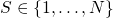:The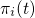are the time-dependent occupation probabilities, which fulfill the normalization condition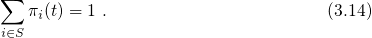This equation states that the probability of finding the system in one of the states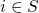equals unity. The time-dependent probability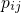for a transition from stateto state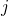can be formulated as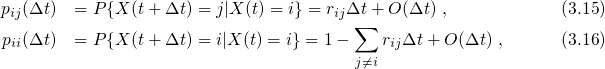where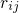denotes the transition rate belonging to. The time evolution of the system can be described by the first-order partial differential equation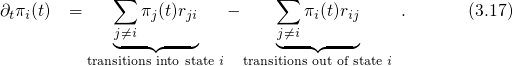The above equation is referred to as the Master or rate equation and controls the time-dependent occupation probabilities. The first term on the right-hand side represents the transitionswhile the second term stands for the opposite direction. With the definition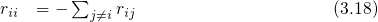the rate equation (3.17) can rewritten as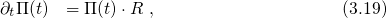where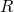denotes the matrix belonging to the elements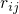. The solution of equation (3.19) is given by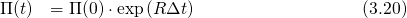and can be expanded into a truncated Taylor series.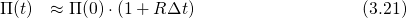Assuming detailed balance, the time derivative of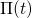vanishes and the equilibrium occupation probabilities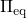must satisfy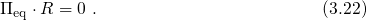In the case of charge trapping, a single defect is represented by one vector. When considering a large ensemble of defects, the stochastic behavior vanishes and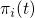andcan be replaced by their corresponding expectation values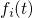and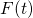, respectively. Then the equations (3.19) and (3.22) readand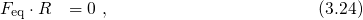respectively.

When applying Markov theory to charge trapping in NBTI, each state must be assigned to a certain configuration and a certain charge state of a defect. The rateslinking the statesandmust be related to certain defect transitions, such as charge transfer reactions or thermally activated rearrangements of the defect structure. The trapping dynamics are then governed by equation (3.23), which must be solved as a function of time. The details of the applied numerical procedures are outlined in Fig. 3.2.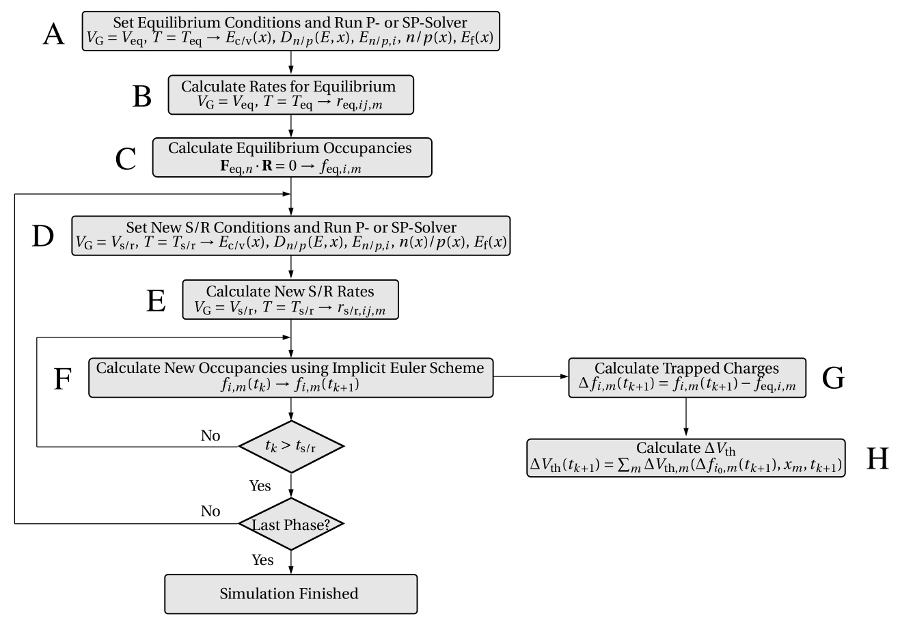Figure 3.2: A flow chart diagram for the calculation of stress/relaxation curves. At first, the occupations for equilibrium conditions (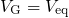,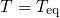) are calculated in steps A, B, and C. During one stress or relaxation cycle, the band edges (D) and the transition rates (E) must be determined at first. Then the corresponding the time-dependent occupations (F) and the resulting threshold voltage shift (G,F) are evaluated at each time step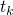. This complete cycle must be performed for each stress or relaxation phase.

Before the real device is subject to stress, the device is assumed to be in equilibrium. The corresponding band diagram is computed by a P/SP-solver (A) for the equilibrium conditions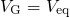and. Based on this information, the transition rates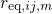(B) can be evaluated for each defect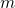. With the rates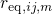at hand, the equilibrium occupation probabilitiesare calculated using the equation (3.24) and must be subsequently stored (C). When stress sets in, the gate voltage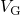and the temperature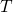are changed to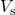and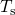, respectively. Since this alters the band diagram and in consequence the transition rates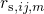, the S/SP-solver step (D) and the calculation of the rates (E) must be repeated. Then the implicit Euler method is employed for the numerical time integration of equation (3.23). This iteration scheme must be continued until the end of the stress time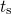has been reached. At each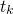, the change in the defect occupancies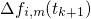(G) and the corresponding threshold voltage shift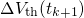(H) are computed. With the beginning of the relaxation phase,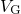andare modified again and the whole iteration loop including the steps D, E, F, G, and H must be repeated. This can be continued for several stress/relaxation cycles with different stress/relaxation conditions. The steps E, F, and G of numerical procedure in Fig. 3.2 must be repeated for each individual defect, where the calculation of the rates requires most of the computation time.

For the evaluation of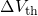, the charge sheet approximation is employed.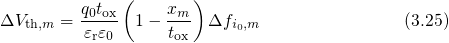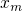and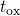are the trap depth and the thickness of the dielectric, respectively. The above expression gives the threshold voltage shift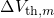due to a single trapin the ‘charged’ state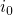. It is noted that the term in the parentheses of equation (3.25) accounts for the trap depth dependence of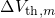according to which traps closer to the interface have a larger impact on the threshold voltage. However, there also exists a strong dependence on the lateral location of the traps, totally neglected in the above equation. Recall in this context that the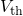steps in the TDDS measurements have also been caused by single charged traps. The wide variations of these steps have been ascribed to the position of the traps relative to the current percolation path and follow an exponential distribution according to an investigation carried out by Kazcer et al.  . This issue has been intensively studied under the name random dopant fluctuations  and gained large interest due to its serious influence on the lifetime projection. For instance, the charging of one defect can even produce step heights in the threshold voltage beyond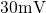, which already violates typical lifetime criteria. By contrast, the charge sheet approximation rests on the assumption that a trapped charge is distributed over the whole plane parallel to the interface. Therefore, variations in the threshold voltage shift due to random dopant fluctuations remain unconsidered in this approximation.

Small-area devices often contain only a handful of defects. Then the steps B, C, E, F, G, and H can be performed at computationally feasible costs. But since the calculation time increases with the number of defects, the simulation of large-area devices can become time-consuming. In order to reduce computation time for these simulations, a certain number of defects with similar properties are grouped together and replaced by one representative trap. This approach implies that the channel area covers several defects with similar properties including the trap level and the spatial location among others1. Furthermore, it is important to note here that the value of trap density is often quite inaccurate and can differ by some orders of magnitude. Therefore, the number of traps must be treated as a variable in the simulations. This means that the calculated degradation curves can be scaled to the experimental data in order to achieve reasonable agreement of the simulations with the measurements.